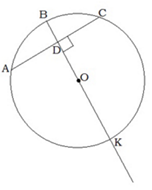• The learning process is entertaining and exciting
• The solved tasks turn into coordinates
• Along with your schoolmates and friends, go on an adventure, searching for hideouts
• E-mail:

# 3073.3. Diameter, which is perpendicular to chord, properties.

#### Calculate the AC, if AD is 12cm and BK is 26cm!cm

#### Next task: 3078. Diameter, which is perpendicular to chord, properties.

8
Subject
Geometry
Theme
Circle, center angle and indrawn angle.
Subtheme
Diameter, which is perpendicular to chord, properties.

#### Solve tasks to find hidden Geo point coordinates

1. Algebra: Square root properties
2. Geometry: Pythagorean theorem. Right angled triangle signs.
3. Physics: Pressure.
4. Geometry: Circle in a triangle, centers location.
5. Geometry: Trapeze, elements, trapeze types, trapeze properties, isosceles trapeze signs.
6. Algebra: Numerical approximation.
7. Geometry: Diameter, which is perpendicular to chord, properties.
8. Algebra: Square trinomial roots
9. Geometry: Rectangular (S = ab, a, b - edges) and area of the square (S=a^2 , a - edge) formula.
10. Algebra: ax² + bx = 0